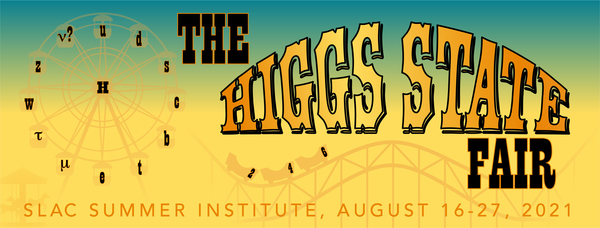#49th SLAC Summer Institute (SSI 2021)

16-27 August 2021
SLAC
America/Los_Angeles timezone

## On Next-to-Soft Resummation to Inclusive Cross-sections and Rapidity distributions

Not scheduled
20m
ZOOM videoconf (SLAC)

### Speaker

POOJA MUKHERJEE (The Institute of Mathematical Sciences)

### Description

We present a formalism that resums threshold enhanced large logarithms to all orders in perturbation theory for the production of a pair of leptons in Drell-Yan process and of Higgs boson in gluon fusion as well as in bottom quark annihilation. As for the inclusive cross-section, the rapidity distribution is a prime candidate to benefit from threshold resummation, hence we apply the same formalism to rapidity distributions for the aforementioned processes as well. We use collinear factorization and renormalization group invariance to achieve this.
We find that the resummed result is a solution to Sudakov type differential equation and hence it can predict soft plus virtual (SV) contributions as well as next to SV (NSV) contributions to all orders in the strong coupling constant.
The $z$ space resummed result is shown to have an integral representation which allows us to resum the large logarithms of the form $\log^i(N)$ retaining $1/N$ corrections resulting from NSV terms. We also show that in $N$ space, tower of logarithms $a_s^n/N^\alpha \log^{2n-\alpha} (N), a_s^n/N^\alpha \log^{2n-1-\alpha}(N) \cdots$ etc for $\alpha =0,1$ are summed to all orders in $a_s$.

### Primary authors

POOJA MUKHERJEE (The Institute of Mathematical Sciences) Ms Ajjath A. H. (The Institute of Mathematical Sciences) Prof. Ravindran V. (The Institute of Mathematical Sciences) Ms Aparna Sankar (The Institute of Mathematical Sciences) Ms Surabhi Tiwari (The Institute of Mathematical Sciences)